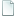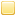##Topic: Two ways of the doing same thing - why does only one of them work? (Read 1355 times)previous topic - next topic

0 Members and 1 Guest are viewing this topic.
• Rhuan
•Two ways of the doing same thing - why does only one of them work?
##### May 16, 2017, 08:26:57 pm
EDIT: I just tested this again and both options now work - so either I changed it whilst pasting it to here and back or the fact that miniSphere now handles transparencies in cloned surfaces correctly has fixed it.

I can't figure this one out, as far as I can see these two pieces of code for reading a Tiled JSON map file and drawing each layer of the map onto the screen should be functionally identical and yet one worked perfectly (except for minisphere not handling the alpha channel) and the other worked for 1 layer and produced garbage for the rest - I used drawText to output the various increment/index values and coordinates and they were all correct all the way through in both cases can anyone see any reason why the first script would not work?

So this version did not work - it would do layer 0 correctly it would produce total garbage for any other layer, including if I adjusted K to start on a different layer.
Code: [Select]
`function Load_map(name){  var m_file = OpenRawFile("../maps/"+name+".json", false);  var m_data = CreateStringFromByteArray(m_file.read(m_file.getSize()));  var m_raw = JSON.parse(m_data);  var t_surface = LoadSurface("../maps/"+m_raw.tilesets.image);  var m_layers = [];  var temp_x = 0;  var temp_y = 0;      for(var i = 0, j = 0, k = 0, l = 0; k < m_raw.layers.length; ++ k, l = 0)  {    m_layers[k] = CreateSurface(m_raw.layers[k].width * m_raw.tilesets.tilewidth, m_raw.layers[k].height * m_raw.tilesets.tileheight,CreateColor(0,0,0,0));    for(i = 0; i < m_raw.layers.height; ++ i)    {      for(j = 0; j < m_raw.layers.width; ++ j, ++l)      {        temp_x = Math.max(0,(m_raw.layers[k].data[l] - 1) % m_raw.tilesets.columns);        temp_y = Math.max(0,(m_raw.layers[k].data[l] - 1 - temp_x) / m_raw.tilesets.columns);        m_layers[k].blitSurface(t_surface.cloneSection(temp_x * 32, temp_y * 32, 32, 32), j*32, i * 32);      }    }    m_layers[k].blit(0,0);    FlipScreen();    GetKey();  }}`

This version worked perfectly.
Code: [Select]
`function Load_map(name){  var m_file = OpenRawFile("../maps/"+name+".json", false);  var m_data = CreateStringFromByteArray(m_file.read(m_file.getSize()));  var m_raw = JSON.parse(m_data);    var t_surface = LoadSurface("../maps/"+m_raw.tilesets.image);  var m_layers = [];  var temp_x = 0;  var temp_y = 0;  var t = 1;  var tiles = [];    tiles = t_surface.cloneSection(0, 0, 32, 32);  for(temp_y = 0; temp_y < 52; ++temp_y)  {    for(temp_x = 0; temp_x < 20; ++temp_x, ++t)    {      tiles[t] = t_surface.cloneSection(temp_x * 32, temp_y * 32, 32, 32);      }  }  for(var i = 0, j = 0, k = 0, l = 0; k < m_raw.layers.length; ++ k, l = 0)  {    m_layers[k] = CreateSurface(m_raw.layers[k].width * m_raw.tilesets.tilewidth, m_raw.layers[k].height * m_raw.tilesets.tileheight,CreateColor(0,0,0,0));    for(i = 0; i < m_raw.layers.height; ++ i)    {      for(j = 0; j < m_raw.layers.width; ++ j, ++l)      {        m_layers[k].blitSurface(tiles[m_raw.layers[k].data[l]], j*32, i * 32);      }    }    m_layers[k].blit(0,0);    FlipScreen();    GetKey();  }}`